Share

# Frank solutions for ICSE Class 10 Physics Part 2 chapter 5 - Heat [Latest edition]

Course

#### Chapters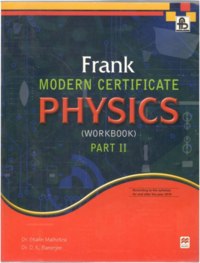## Chapter 5: Heat

Exercise 5.1ExerciseExercise 5.2

### Frank solutions for ICSE Class 10 Physics Part 2 Chapter 5 Heat Exercise 5.1 [Page 233]

Exercise 5.1 | Q 1 | Page 233
What do you mean by thermal energy?
Exercise 5.1 | Q 2 | Page 233
Is heat a form of energy?
Exercise 5.1 | Q 3 | Page 233
What do you understand by the term 'temperature'?
Exercise 5.1 | Q 5 | Page 233
What is the SI unit of heat and temperature?
Exercise 5.1 | Q 6 | Page 233
Define 1 joule.
Exercise 5.1 | Q 7 | Page 233

Which is bigger: 1 joule or 1 calorie?

Exercise 5.1 | Q 8 | Page 233
Name the instrument used to measure temperature.
Exercise 5.1 | Q 9.1 | Page 233

Plot a graph taking oC on the Y-axis and the corresponding oF along the X-axis.

Exercise 5.1 | Q 9.2 | Page 233

Plot a graph taking oF along the Y-axis and the corresponding oC on the X-axis.

Exercise 5.1 | Q 10 | Page 233
Name the physical quantity that measures the degree of hotness.
Exercise 5.1 | Q 11 | Page 233
A substance is heated gradually. Does its energy increase or decrease?
Exercise 5.1 | Q 12 | Page 233
Why do the molecules of a gas move about freely?
Exercise 5.1 | Q 13 | Page 233
Name the two scales used for measuring temperature.
Exercise 5.1 | Q 14 | Page 233
What kind of thermometer is commonly used?
Exercise 5.1 | Q 15 | Page 233
What is the other name of Doctor's thermometer?
Exercise 5.1 | Q 16 | Page 233
Express the melting point of ice on the Celsius scale and the Fahrenheit scale.
Exercise 5.1 | Q 17 | Page 233
Which scale of temperature is commonly used and why?
Exercise 5.1 | Q 18 | Page 233
What is the normal body temperature of a healthy person on the Celsius scale?
Exercise 5.1 | Q 19 | Page 233
Write down a relationship to convert temperature on the Fahrenheit to Celsius scale.
Exercise 5.1 | Q 20 | Page 233
What are the upper and lower fixed points on the Fahrenheit scale?
Exercise 5.1 | Q 21 | Page 233
Describe the Celsius scale of temperature.
Exercise 5.1 | Q 22 | Page 233
Which represents a greater temperature rise, 200C or 200 F?
Exercise 5.1 | Q 23 | Page 233

Express -400C on the Fahrenheit scale.

Exercise 5.1 | Q 24 | Page 233
Express 2120F on the Celsius scale.

### Frank solutions for ICSE Class 10 Physics Part 2 Chapter 5 Heat Exercise 5.1, Exercise [Pages 229 - 234]

Exercise 5.1 | Q 1 | Page 234

Express the temperature of 0 K on the:
(a) Celsius scale
(b) Fahrenheit scale

Exercise 5.1 | Q 2 | Page 234
What is absolute zero?
Exercise 5.1 | Q 3 | Page 234
If the temperature of a body is 200C, what will be the corresponding temperature on the Kelvin scale?
Exercise 5.1 | Q 4 | Page 234
Convert 370C temperature to the Fahrenheit scale.
Exercise 5.1 | Q 5 | Page 234

Write the SI unit of:
(i) Amount of heat
(ii) Heat capacity
(iii) Specific Heat capacity

Exercise 5.1 | Q 6 | Page 234
A bucket contains 8 kg of water at 250 C. 2 kg of water at 800 C is poured into it. Neglecting the heat energy absorbed by the bucket, calculate the final temperature of water.
Exercise | Q 6 | Page 229
Write the SI unit of latent heat.
Exercise 5.1 | Q 7 | Page 234
What mass of a liquid A of specific heat capacity 0.84 J K-1 and at a temperature 400C must be mixed with 100 g of a liquid B of specific heat capacity 2.1 J g-1 K-1 and at 200C, so that final temperature of mixture becomes 320C?
Exercise 5.1 | Q 8 | Page 234
Write the approximate value of specific heat capacity of water in SI unit.
Exercise 5.1 | Q 9 | Page 234
The sp. Heat capacity of water is 4200 J kg-1 K-1. What information does this convey?
Exercise 5.1 | Q 10 | Page 234

Give one example each where high specific heat capacity of water is used (i) In cooling, (ii) As heat reservoir.

Exercise 5.1 | Q 11 | Page 234
What is a calorimeter? Why is it made of copper? Give two reasons.
Exercise 5.1 | Q 12 | Page 234
A piece of iron of mass 2.0 kg has a thermal capacity of 966 J/0C. Find: (i) Heat energy needed to warm it by 150C, and (ii) Its specific heat capacity in SI unit.
Exercise 5.1 | Q 13 | Page 234
Why do the farmers fill their fields with water on a cold winter night?
Exercise 5.1 | Q 14 | Page 234
The temperature of 600 g of cold water rises by 150C when 300 g of hot water at 500C is added to it. What was the initial temperature of the cold water?
Exercise 5.1 | Q 15 | Page 234

Define the term 'heat capacity' and state its unit.

Exercise 5.1 | Q 16 | Page 234

Define the term 'specific heat capacity' and state its unit.

Exercise 5.1 | Q 17 | Page 234

0.5 kg of lemon squash at 300C is placed in a refrigerator which can remove heat at an average rate of 30 Js-1. How long will it take to cool the lemon squash to 50C?(Sp. heat capacity of lemon squash = 4200 J kg-1 oC-1.)

Exercise 5.1 | Q 18 | Page 234
Describe a method to determine the specific heat capacity of a solid, like a piece of copper.
Exercise 5.1 | Q 19 | Page 234
Discus the role of high specific heat capacity of water with reference to climate in coastal areas.
Exercise 5.1 | Q 20 | Page 234
State the principle of calorimetry.
Exercise 5.1 | Q 21 | Page 234
Water is used as an effective coolant. Give reason.

### Frank solutions for ICSE Class 10 Physics Part 2 Chapter 5 Heat Exercise 5.2 [Page 241]

Exercise 5.2 | Q 1 | Page 241
Define specific heat. Is it same as heat capacity?
Exercise 5.2 | Q 2 | Page 241
Write the SI unit of specific heat.
Exercise 5.2 | Q 3 | Page 241
Name the physical quantity which has its unit as j/(kg)/(K).
Exercise 5.2 | Q 4 | Page 241
State the principle of calorimetry.
Exercise 5.2 | Q 5 | Page 241
What do you mean by thermal capacity?
Exercise 5.2 | Q 6 | Page 241

The product of mass and specific heat is known as ..........

Exercise 5.2 | Q 7 | Page 241
Does the specific heat depend upon the temperature?
Exercise 5.2 | Q 8 | Page 241
Why does a copper rod become warmer than an aluminium rod of the same mass, when put in the sun for the same length of time?
Exercise 5.2 | Q 9 | Page 241

The amount of heat required to raise the temperature of a body by 10C is called .........

Exercise 5.2 | Q 10 | Page 241
Name the physical quantity which has its unit as J/0C.
Exercise 5.2 | Q 11 | Page 241
What is the value of specific heat of water?
Exercise 5.2 | Q 12 | Page 241

The substances like water which have ........... Heat capacity warm up more slowly than substances like iron which have .......... heat capacity.

Exercise 5.2 | Q 13 | Page 241
What do you mean by latent heat?
Exercise 5.2 | Q 14 | Page 241
Write the SI unit of latent heat.
Exercise 5.2 | Q 15 | Page 241

What do you mean by the statement?
'The specific latent heat capacity of fusion of ice is 336 J per g'?

Exercise 5.2 | Q 16 | Page 241

When a liquid is solidified, it many either expand or contract. As water freezes to form ice it ........... And .............. in volume by ............. per cent.

Exercise 5.2 | Q 17 | Page 241
What is the effect of impurities on the melting point of ice?
Exercise 5.2 | Q 18 | Page 241
What is the effect of pressure on the melting point of ice?
Exercise 5.2 | Q 19 | Page 241
What do you mean by regelation?
Exercise 5.2 | Q 20 | Page 241
What do you understand by the latent heat of vaporization?
Exercise 5.2 | Q 21 | Page 241
What is the effect of pressure on the boiling point of water?
Exercise 5.2 | Q 22 | Page 241
What is the latent heat of fusion of ice?
Exercise 5.2 | Q 23 | Page 241
What is the latent heat of vaporization of steam?
Exercise 5.2 | Q 24 | Page 241

The physical quantity which does not change during change of state is ............. of the body.

Exercise 5.2 | Q 25 | Page 241
Why does ice appear colder than water at 0°C?
Exercise 5.2 | Q 26 | Page 241
Why does cause more severe burns than water at 1000C?
Exercise 5.2 | Q 27 | Page 241

What is the unit of heat capacity in CGS system?

Exercise 5.2 | Q 28 | Page 241

1 cal g-1 °C-1 = ........... J kg-1 °C-1.

Exercise 5.2 | Q 29 | Page 241
The specific heat of body is 0.2 cal g-1 °C-1. What is the meaning of this statement?
Exercise 5.2 | Q 30 | Page 241
The specific heat of a substance of mass 100 g is 0.04 cal g-1 0C-1. What is its heat capacity?
Exercise 5.2 | Q 31 | Page 241
Write down the relation between specific heat and heat capacity.
Exercise 5.2 | Q 32 | Page 241
Does specific heat depend upon the mass of a substance?
Exercise 5.2 | Q 33 | Page 241
How much is the specific heat of water in SI units?
Exercise 5.2 | Q 34 | Page 241
Name the substance that has the maximum value of specific heat.
Exercise 5.2 | Q 35 | Page 241
State the factors on which heat gained or lost by a substance depends.
Exercise 5.2 | Q 36 | Page 241
Why is the ocean known as storehouse of heat energy?
Exercise 5.2 | Q 37 | Page 241
Why do we use water as a cooling agent in the radiators of automobiles?

### Frank solutions for ICSE Class 10 Physics Part 2 Chapter 5 Heat Exercise 5.2 [Page 242]

Exercise 5.2 | Q 1 | Page 242
What is meant by change of state?
Exercise 5.2 | Q 2 | Page 242
State whether heat energy is absorbed or released during melting of ice.
Exercise 5.2 | Q 3 | Page 242
State whether heat energy is absorbed or released during freezing of ice.
Exercise 5.2 | Q 4 | Page 242

Temperature ............ When ice melts at 0°C.

Exercise 5.2 | Q 5 | Page 242
Why does the temperature of a substance remain constant during the process of melting?
Exercise 5.2 | Q 6 | Page 242
Why does the temperature of a substance remain constant during the process of boiling?
Exercise 5.2 | Q 7 | Page 242
Atmosphere is usually warm during snowfall. Why?
Exercise 5.2 | Q 8 | Page 242
Find the amount of heat required to convert 2 g of ice at 0°C into water at 0°C. Latent heat of fusion of ice= 336 Jg-1.
Exercise 5.2 | Q 9 | Page 242

Find the amount of heat required to convert 100 g of water at 1000C into steam at 100°C. Latent heat of vapourisation of water is 540 calg-1.

Exercise 5.2 | Q 10 | Page 242
It is much easier to skate on rough ice than on glass. Give reasons.
Exercise 5.2 | Q 11 | Page 242
Bottled drinks are cooled more effectively when surrounded by lamps of ice than by cold water at 00C. Why?
Exercise 5.2 | Q 12 | Page 242

A substance is heated at a constant rate from a low temperature to a high temperature. A graph of temperature against time is shown in the figure. Which part or parts of the graph correspond(s) to the substance existing in two states?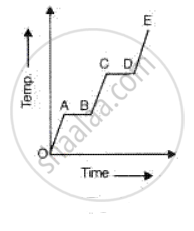Exercise 5.2 | Q 13 | Page 242

A solid is heated at a constant rate in an insulated container till half of it vaporizes. Which of the following graphs show the temperature changes during this time?

•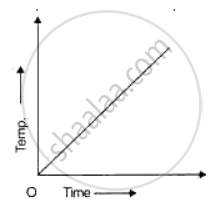•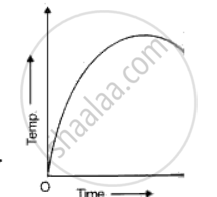•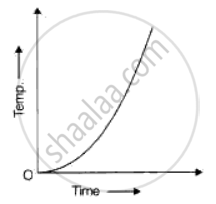•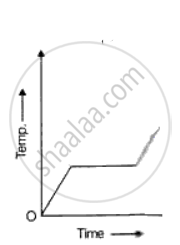•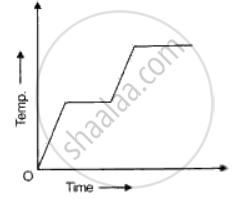Exercise 5.2 | Q 14 | Page 242

The graph given below represents a cooling curve fore a substance being cooled from a higher temperature to a lower temperature.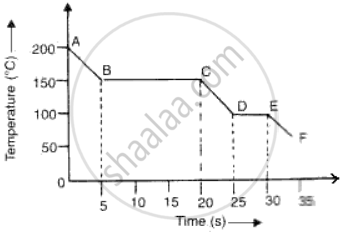(a)What is the boiling point of the substance?
(b)What happens in the region DE?
(c)What is the melting point of the substance?

### Frank solutions for ICSE Class 10 Physics Part 2 Chapter 5 Heat Exercise 5.2 [Page 243]

Exercise 5.2 | Q 1 | Page 243

A slab of ice at -50°C is constantly heated till the steam attains a temperature of 150°C. Draw a graph showing the change of temperature with time. Label the various parts of graph properly.

Exercise 5.2 | Q 2 | Page 243

A piece of ice is heated at a constant rate. The variation in temperature with time of heating is shown in the graph (see fig.)
(i) What is represented by AB?
(ii) What does CD represent?
(iii) What conclusion can you draw regarding the nature of ice from the graph below?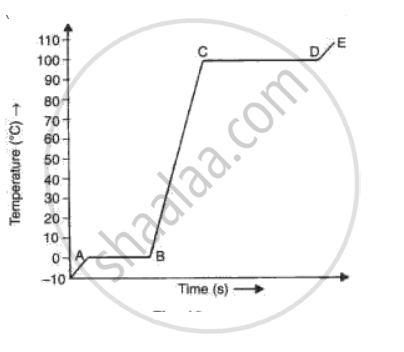### Frank solutions for ICSE Class 10 Physics Part 2 Chapter 5 Heat Exercise [Page 245]

Exercise | Q 1.1 | Page 245

Make the correct choice in each of the following:
(a) In what unit is the specific heat capacity expressed?

• J

• J/kg°C

• J/kg

• kg/J°C

• kg/J

Exercise | Q 1.2 | Page 245

(b) 2000 J of heat energy is required to raise the temperature of 4 kg of a
metal by 3°c. Which expression gives the specific heat capacity of the metal?

• 2000/( 4x3) J/kg°C

• 2000/4 x 4 Jjkg°C

• 2000 x 4/3 J/kg°C

• 4 x 3/2000 J/kg°C

• 2000 x 3/4 J/kg°C

Exercise | Q 1.3 | Page 245

A substance is heated at a constant rate from a low temperature to a high
temperature. A graph of temperature against time is drawn and is shown in
fig. . Which part of parts of the graph corresponds to the substance existing
in two states?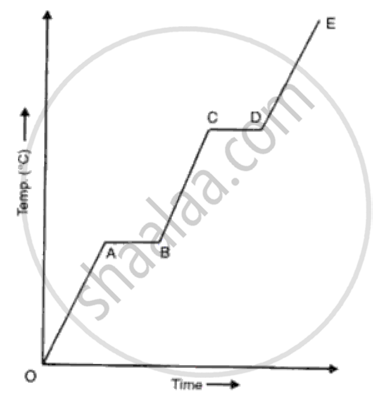• All of it

• OA, BC and DE

• AB and CD

• DE

• CB

Exercise | Q 1.4 | Page 245

What change in heat energy occurs when lead at its melting point
solidifies without change in the temperature?

• Latent heat is emitted.

• Latent heat is absorbed.

• Specific heat capacity is emitted.

• Specific heat capacity is absorbed.

• Both latent heat and specific heat are emitted.

Exercise | Q 1.5 | Page 245

A solid is heated at a constant rate in an insulated container till half of it
vapourises. Which graph in the following fig shows the temperature changes during this time?

•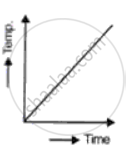•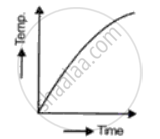•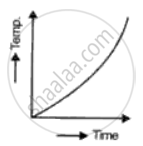•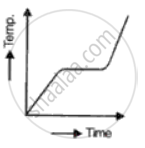•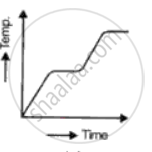### Frank solutions for ICSE Class 10 Physics Part 2 Chapter 5 Heat Exercise [Page 246]

Exercise | Q 1.1 | Page 246

Define heat capacity and state its units.

Exercise | Q 1.2 | Page 246

What amount of heat would be required to raise the temperature of 500 g of water from 30°C to 50°C? (sp. heat cap. of water= 4200 J/kg°C)

Exercise | Q 2.1 | Page 246

Sp. heat capacity of copper is 390 J/kg°C. What do you understand by this statement?

Exercise | Q 2.2 | Page 246

Calculate the amount of heat given out by 600 g of aluminium while cooling from 100oC to 30°C. (Sp. heat cap. of aluminium = 900 J/kgK.)

Exercise | Q 3.1 | Page 246

State the principle of calorimeter and define calorie and kilocalorie.

Exercise | Q 3.2 | Page 246

400 g of water at 80°C is mixed with 1 kg of water at 20°C. If the sp. heat cap. of water is 4200 J/kgK, find the final temperature of the mixture.

Exercise | Q 3.3 | Page 246

A burner raises the temperature of 360 g of water from 40°C to 100°C in 5 minutes. Calculate the rate of heat supplied by the burner.

Exercise | Q 4 | Page 246

A solid of mass 80 g at 80°C is dropped in 400 g water at 10°C. If final temp. is 30°C, find the sp. heat cap. of the solid.

Exercise | Q 5 | Page 246

A mass of 200 g of mercury at 100°C is poured into water at 20°C. If the final temperature of the mixture is 25°C, find the mass of water. (Sp. heat cap. of mercury is 140 J/kgK).

Exercise | Q 6 | Page 246

A piece of copper of mass 1 kg is dropped into 2 kg of water at 15°C. If the final temperature of the mixture is 40°C, calculate the intial temperature of copper.

Exercise | Q 7 | Page 246

A copper calorimeter of heat capacity 32 J/°C contains 100 g of oil at 30°C. 80 g of a metal of sp. heat cap. 0.12 J/g°C at 90°C is dropped into the oil and final temperature is 35°C. Calculate the sp. heat cap. of the oil.

Exercise | Q 8.1 | Page 246

Define the following terms:
(i) Latent heat,
(ii) Latent heat of fusion of ice.

Exercise | Q 8.2 | Page 246

Calculate the amount of heat required to change 40 G of ice at -10°C into water at 20°C. (sp. heat cap. of ice = 2.1 J/g°C, latent heat of fusion of ice = 330 j/g)

Exercise | Q 9.1 | Page 246

Define the following terms:
(i) Specific latent heat,
(ii) Specific latent heat of fusion.

Exercise | Q 9.2 | Page 246

What do you understand by the statement sp. latent heat of vapourisation of steam is 2268 J/kg?

Exercise | Q 9.3 | Page 246

Calculate the amount of heat given out while converting 100 g of water at 50°C into ice at -5°C. (refer Q.9 for data).

Exercise | Q 10 | Page 246

When steam at 100°C is passed through a hole drilled in a slab of ice at 0°C certain amount of ice melts. Find the amount of ice that melts. (sp. latent heat of fusion of ice = 336 J/g, sp. latent heat of vapourisation of steam = 2268 J/g, Sp. heat cap. of water= 4.2 J/g°C) (Take mass of steam as 1000 g)

Exercise | Q 11 | Page 246

A slab of ice at -5°C is constantly heated till it changes to steam at 105°C. Draw a graph showing the change of temperature with time and label the different parts of the graph.

Exercise | Q 12 | Page 246

200 g of of ice at 0°C is added to 2 kg of water at 30°C. What is the temperature of the mixture?

Exercise | Q 13 | Page 246

A solid of mass 100 g is heated with a burner which is supplying heat at the rate of 100 J/s. The graph in the following fig shows the changes that take place.
(a) What is the melting point of the substance?
(b) What is boiling point of the substance?
(c) Calculate the Sp. heat cap. of solid.
(d) Calculate the Sp. latent heat of fusion.
(e) Calculate the Sp. heat cap. of the liquid.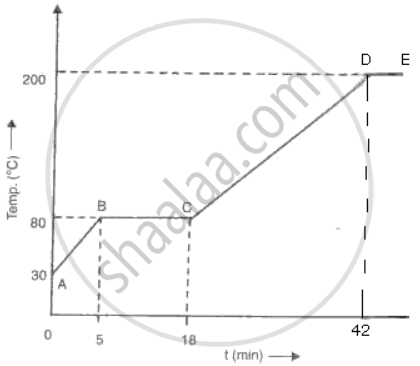### Frank solutions for ICSE Class 10 Physics Part 2 Chapter 5 Heat Exercise [Page 247]

Exercise | Q 1 | Page 247

1 kg of molten lead at its melting point of 327°C is dropped in 1 kg of water at 20°C. Assuming no heat is lost; calculate the final temperature of water. (Sp. heat cap. of lead = 130 J/kg°C, Sp. latent heat of fusion of lead = 27000 J/g, Sp. heat cap. of water = 4200 j/kg°C)

Exercise | Q 2 | Page 247

How much steam at 100oC must be passed into 120 g of water at 20oC to raise the temperature to 40oC?

Exercise | Q 3 | Page 247

Heat is supplied at a constant rate to 200 g of ice at 0°C until finally all the ice is converted into steam at 100°C. If the specific latent heat of ice is 3.3 x 105 J/kg and that of steam is 2.3 x 106 J/kg and the Sp. heat capacity of water is 4200 J/kg, draw rough graphs for the following to illustrate the changes which take place as the solid ice is converted into water and finally into steam, the pressure remaining constant all the time.
(a) Volume against temperature,
(b) Temperature against time.

Exercise | Q 4.1 | Page 247

Give scientific reasons for the following:
It is much easier to skate on rough ice than on glass.

Exercise | Q 4.2 | Page 247

Give scientific reasons for the following:

Sand mixed with salt is often spread over the icy roads in winter.

Exercise | Q 4.3 | Page 247

Give scientific reasons for the following:

A steam burn is usually worse than a hot water burn.

Exercise | Q 4.4 | Page 247

Give scientific reasons for the following:

Bottled drinks are cooled more effectively when surrounded by lumps of ice than by cold water at 0°.

Exercise | Q 5 | Page 247

A bucket contains 10 liters of water at 80°C. Cold water at 25°C is run from a tap into the bucket of hot water for 20 seconds and the temp. Of the water in the bucket falls to 50°C.
(a) Calculate the rate at which cold water came out of the tap.
(b) State an assumption made in the above calculation.

Exercise | Q 6 | Page 247

650 J of heat is required to raise the temp. of 0.25 kg of lead from 15°C to 35°C. Calculate the Sp. heat capacity of lead.

Exercise | Q 7 | Page 247

A copper calorimeter weighing 57.5 g contains 60 g of water at 12°C. 55 g of iron nails at 100°C are dropped into the calorimeter ands stirred rapidly. The final temperature attained by the calorimeter and its contents is 20°C. Calculate the specific heat of iron. (Sp. heat of copper = 0.4 J/g°C, Sp. heat of water = 4.2 J/g°C).

Exercise | Q 8 | Page 247

1 kg of molten lead at its melting point of 327°C is dropped into 1 kg of water at 20°C. Assuming no loss of heat, calculate the final temp. of water. (Sp. heat of lead = 130 J/kg°C, latent heat of lead = 27000 J/kg and Sp. heat of water = 4200 J/kg°C).

Exercise | Q 9 | Page 247

Some heat is given to 120 g of water and its temp. rises by 10 K. When the same amount of heat is given to 60 g of oil, its temp. rises by 40 K. The Sp. heat of water is 4200 J/kgK. Calculate:
(a) The amount of heat in joules given to water,
(b) The specific heat capacity of the oil.

Exercise | Q 10 | Page 247

A block of lead of mass 250 g, at 27°C was heated in a furnace till it completely melted. Find the quantity of heat required
(a) To bring the lead block upto its melting point,
(b) To completely melt the block at its melting point (Melting point of lead is 327°C, Sp. heat cap. of lead is 0.13 J/gk and latent heat of fusion of lead is 26 J/g)

Exercise | Q 11 | Page 247

100 g of ice at -10°C is heated. It is converted into steam. Calculate the quantity of heat which it has consumed. (Sp. heat of ice = 2100J/kg°C, sp. heat of water= 4200 J/kgK, sp. heat of water = 42000 J/kgK, sp. latent heat of ice = 2260000 J/kg).

Exercise | Q 12 | Page 247

A refrigerator converts 100 g of water at 20°C to ice at -10°C in 73.5 minutes. Calculate the average rate of heat extraction from water in watt. (Sp. heat of ice= 2100 J/kgK, sp. heat of water = 4200 J/kgK, Sp. latent heat of ice = 336000 J/kg.)

Exercise | Q 13 | Page 247

(i) A molten metal weighing 150 g is kept at its melting point 800°C. When it is allowed to solidify at the same temp, it gives out 75000 J of heat. What is the specific heat of the metal?

(ii) If its specific heat capacity is 200 J/kgK? How much additional heat will it give out in cooling to -50°C?

Exercise | Q 14 | Page 247

40 g of ice at -16°C is dropped into water at 0°C, when 4 g of water freezes into ice. If specific heat capacity of ice is 2100 J/kg°C, what will be the latent heat of fusion of ice?

Exercise | Q 15 | Page 247

Calculate the time taken by an immersion heater, which supplies energy at the rate of 7000 J/minute to raise temp. of 5 kg water from 22°C to 47°C.

Exercise | Q 16 | Page 247

In an experiment. 17 g of ice is used to bring down the temp. of 40 g of water from 34°C to its freezing point. The sp. heat capacity of water is 4.25 J/g°C. Calculate sp. latent heat of ice.

Exercise | Q 17 | Page 247

What mass of ice at 0°C will be required to cool 0.9 kg of water from 35°C to 0°C? Assume all the ice used melts. The specific heat capacity of water is 4.2 x 103 J/kgoC and specific latent heat of fusion of ice is 336 x 103 J/kg.

### Frank solutions for ICSE Class 10 Physics Part 2 Chapter 5 Heat Exercise [Page 248]

Exercise | Q 1 | Page 248

State, with reason, which of the two, boiling water or steam both at 100°C will produce more severe burns.

Exercise | Q 2 | Page 248

Ice cream appears colder to the mouth than water at 0°C. Give a reason.

Exercise | Q 3 | Page 248
Why do bottled soft drinks get cooled, more quickly, by the ice cubes than by the iced water?
Exercise | Q 4 | Page 248

If 10125 J of heat energy boils off 4.5 g of water at 100°C  to steam at 100°C, find the specific latent heat of steam.

Exercise | Q 5.01 | Page 248

Fill in the following blank using suitable word:
SI unit of heat is .........

Exercise | Q 5.02 | Page 248

Fill in the following blank using suitable word:

1 cal = .......... J

Exercise | Q 5.03 | Page 248

Fill in the following blank using suitable word:

Whenever mechanical work is done, .......... Is produced.

Exercise | Q 5.04 | Page 248

Fill in the following blank using suitable word:

Two bodies in contact are said to be in thermal equilibrium, if they have same ...........

Exercise | Q 5.05 | Page 248

Fill in the following blank using suitable word:

The normal temperature of a human body is .............

Exercise | Q 5.06 | Page 248

Fill in the following blank using suitable word:

SI unit of specific heat is ..........

Exercise | Q 5.08 | Page 248

Fill in the following blank using suitable word:

Ice at o0C is colder than ...........at o0C.

Exercise | Q 5.09 | Page 248

Fill in the following blank using suitable word:

Steam at 100oC is hotter than water at ............

Exercise | Q 5.1 | Page 248

Fill in the following blank using suitable word:

Evaporation causes ..........

Exercise | Q 5.7 | Page 248

Fill in the following blank using suitable word:

The amount of heat required to change the state of a physical substance without any change of temperature is called .......... of the substance.

Exercise | Q 6 | Page 248

Which has more heat: 1 g of ice at 0°C or 1 g of water at 0°C? Give reason.

Exercise | Q 7 | Page 248

Which contains more heat: 1 G water at 100°C or 1 g steam at 100°C? Give reason.

Exercise | Q 8 | Page 248

Which requires more heat: 1 g ice at 0°C or 1 g water at 0°C to raise its temperature to 10°C? Explain your answer.

Exercise | Q 9 | Page 248
Write down the approximate temperature at which the water boils in a pressure cooker.

## Chapter 5: Heat

Exercise 5.1ExerciseExercise 5.2## Frank solutions for ICSE Class 10 Physics Part 2 chapter 5 - Heat

Frank solutions for ICSE Class 10 Physics Part 2 chapter 5 (Heat) include all questions with solution and detail explanation. This will clear students doubts about any question and improve application skills while preparing for board exams. The detailed, step-by-step solutions will help you understand the concepts better and clear your confusions, if any. Shaalaa.com has the CISCE ICSE Class 10 Physics Part 2 solutions in a manner that help students grasp basic concepts better and faster.

Further, we at Shaalaa.com provide such solutions so that students can prepare for written exams. Frank textbook solutions can be a core help for self-study and acts as a perfect self-help guidance for students.

Concepts covered in ICSE Class 10 Physics Part 2 chapter 5 Heat are Concept of Calorimetry Numercials:, Concept of Calorimetry, Calorimetry - Specific Heat Capacity, Calorimetry - Principle of Method of Mixtures, Heat - Latent Heat, Heat - Loss and Gain of Heat Involving Change of State for Fusion Only.

Using Frank Class 10 solutions Heat exercise by students are an easy way to prepare for the exams, as they involve solutions arranged chapter-wise also page wise. The questions involved in Frank Solutions are important questions that can be asked in the final exam. Maximum students of CISCE Class 10 prefer Frank Textbook Solutions to score more in exam.

Get the free view of chapter 5 Heat Class 10 extra questions for ICSE Class 10 Physics Part 2 and can use Shaalaa.com to keep it handy for your exam preparation